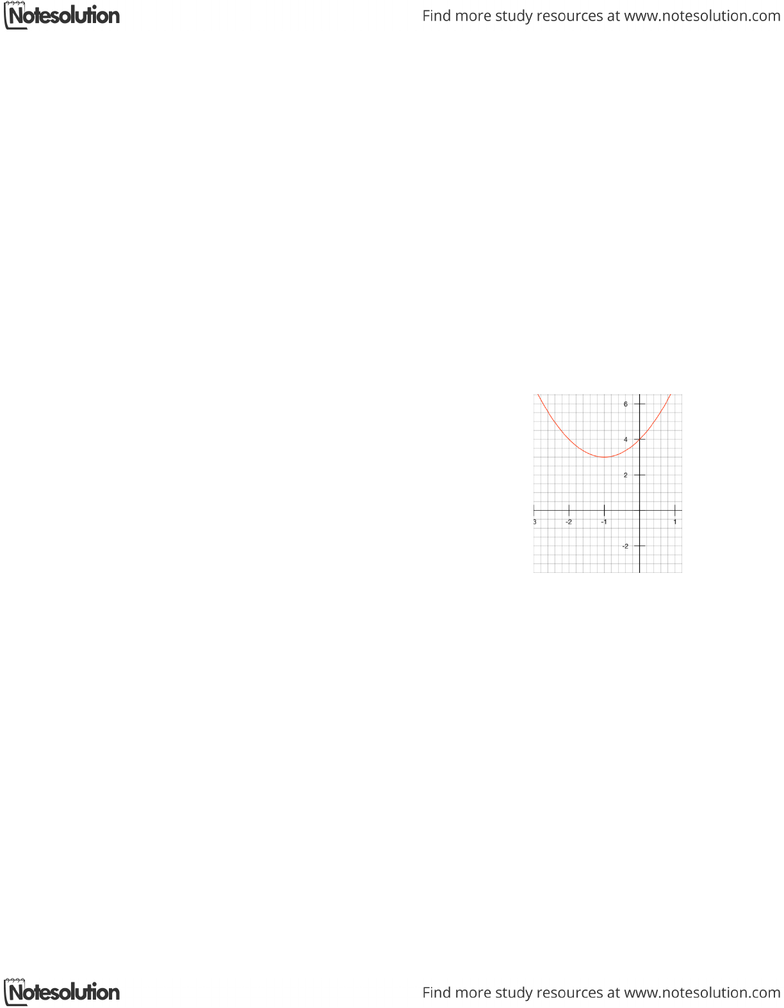Class Notes (1,100,000)
CA (630,000)
UTSG (50,000)
MAT (4,000)
MAT136H1 (900)
all (200)
Lecture

# MAT136H1 Lecture Notes - Polar Coordinate System

Department
Mathematics
Course Code
MAT136H1
Professor
all

Page:
of 110.5 Parametric Equations & Polar Coordinates
Conic Sections
Question #1 (Easy): Vertex, Focus, Directrix for Parabola
Strategy
For a parabola equation  , or   the focus is at   or   and the directrix is
   or  , respectively. The focus can shift horizontal and vertically in the opposite direction of
the numeric values added unto and .
Sample Question
Find the vertex, focus, and directrix of the parabola and sketch the graph.
    
Solution
The equation can be re-written in the form of    ,
which means the vertex is at  and since the parabola is in the form
of  ,   ,   . Then the directrix is     , which
means 3 units down from the vertex. Thus because of the shift of the
vertex, the directrix becomes      . The focus is directly above
the vertex, but moved in the opposite direction, that is in this case
northward by the same unit that directrix is below the vertex. Thus, the
focus is at   